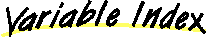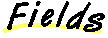`All Packages  Class Hierarchy  This Package  Previous  Next  Index`

# Class juice.operator.ResidualOp

```java.lang.Object
|
+----juice.operator.ResidualOp
```

public class ResidualOp
extends Object
implements Operator
A ResidualOp, R, is defined by an Operator, A, and a Vector in the range of A, call it b, as:

R(x) = A(x) - b

##Ab

##ResidualOp(Operator, Vector)ResidualOp(ResidualOp)

##addImage(Vector, Vector)copy()equals(Object)
Is there any reason to check if two operators are equal?getDomain()getRange()image(Vector)image(Vector, Vector)residual(Vector, Vector, Vector)toString()
Returns a string representation of the object.

##A
``` protected Operator A
```b
``` protected Vector b
```

##ResidualOp
``` public ResidualOp(Operator A,
Vector b)
```ResidualOp
``` protected ResidualOp(ResidualOp src)
```

##getDomain
``` public Space getDomain()
```getRange
``` public Space getRange()
```image
``` public Vector image(Vector d)
```image
``` public void image(Vector d,
Vector r)
```addImage
``` public void addImage(Vector d,
Vector r)
```residual
``` public void residual(Vector d,
Vector b,
Vector r)
```equals
``` public boolean equals(Object obj2)
```
Is there any reason to check if two operators are equal?

Overrides:
equals in class Objectcopy
``` public JamObject copy()
```toString
``` public String toString()
```
Returns a string representation of the object.

Overrides:
toString in class Object

`All Packages  Class Hierarchy  This Package  Previous  Next  Index`